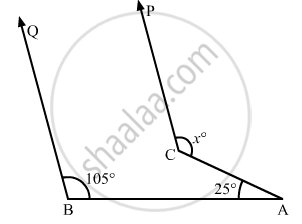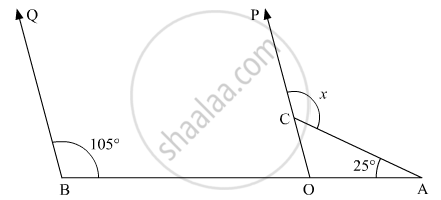# In the Given Figure, If Cp || Dq, Then the Measure of X is - Mathematics

MCQ

In the given figure, if CP || DQ, then the measure of x is• 130°

•  105°

• 175°

• 125°

#### Solution

Let us extend PC to meet AB at point O.It is given that BQ || PO.

Thus,∠QBOand ∠AOP are corresponding angles. Therefore,

∠AOP =  ∠QBO

Given that ∠QBO = 150°, then we have:

∠AOP = 105V

Or,

∠AOC =105°

Also, in ΔAOC, exterior angle is equal to the sum of the interior opposite angles, therefore,

x =  ∠AOC + ∠COA

x = 105° + 25°

x = 130°

Concept: Introduction to Lines and Angles
Is there an error in this question or solution?

#### APPEARS IN

RD Sharma Mathematics for Class 9
Chapter 10 Lines and Angles
Q 30 | Page 57